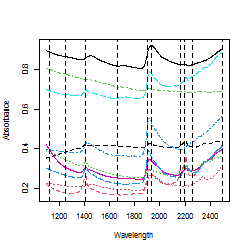Near- and mid-infrared spectroscopy are high–troughput, non–destructive and cheap sensing methods that has a range of applications in agricultural, medical, food and environmental science. A number of R packages of interest for the spectroscopist is already available for processing and analysis of spectroscopic data.

Since the publication of the special special Volume in Spectroscopy and Chemometrics in R [@mullen2007] many spectroscopy-related packages have been released. Most of these packages can be found at the following CRAN task views:

In addition, Bryan Hanson provides a list of Free Open Source Software (FOSS) dedictaed to Spectroscopic applications in gneral (see https://bryanhanson.github.io/FOSS4Spectroscopy/).

## Signal Processing

The aim of spectral pre-treatment is to improve signal quality before modeling as well as remove physical information from the spectra. Applying a pre-treatment can increase the repeatability/reproducibility of the method, model robustness and accuracy, although there are no guarantees this will actually work. The pre-processing functions that are currently available in the package are listed in Table 1.

Table 1. List of pre-processing functions

Function Name Description
movav simple moving (or running) average filter
savitzkyGolay Savitzky–Golay smoothing and derivative
gapDer gap–segment derivative
continuumRemoval compute continuum–removed values
detrend detrend normalization
standardNormalVariate Standard Normal Variate (SNV) transformation
binning average a signal in column bins
resample resample a signal to new band positions
resample2 resample a signal using new FWHM values
blockScale block scaling
blockNorm sum of squares block weighting

We show below how they can be used, using the NIR dataset (NIRsoil) included in the package [@fernandez2008]. Observations should be arranged row-wise.

library(prospectr)
data(NIRsoil)
# NIRsoil is a data.frame with 825 obs and 5 variables: Nt (Total Nitrogen), Ciso
# (Carbon), CEC (Cation Exchange Capacity), train (vector of 0,1 indicating
# training (1) and validation (0) samples), spc (spectral matrix)
str(NIRsoil)
## 'data.frame':    825 obs. of  5 variables:
##  $Nt : num 0.3 0.69 0.71 0.85 NA ... ##$ Ciso : num  0.22 NA NA NA 0.9 NA NA 0.6 NA 1.28 ...
##  $CEC : num NA NA NA NA NA NA NA NA NA NA ... ##$ train: num  1 1 1 1 1 1 1 1 1 1 ...
##  $spc : num [1:825, 1:700] 0.339 0.308 0.328 0.364 0.237 ... ## ..- attr(*, "dimnames")=List of 2 ## .. ..$ : chr  "1" "2" "3" "4" ...
##   .. ..$: chr "1100" "1102" "1104" "1106" ...  ## Noise removal Noise represents random fluctuations around the signal that can originate from the instrument or environmental laboratory conditions. The simplest solution to remove noise is to perform $$n$$ repetition of the measurements, and the average individual spectra. The noise will decrease with a factor $$\sqrt{n}$$. When this is not possible, or if residual noise is still present in the data, the noise can be removed mathematically. ### Moving average or runnnig mean A moving average filter is a column-wise operation which average contiguous wavelengths within a given window size. # add some noise noisy <- NIRsoil$spc + rnorm(length(NIRsoil$spc), 0, 0.001) # Plot the first spectrum plot(x = as.numeric(colnames(NIRsoil$spc)), y = noisy[1, ], type = "l", lwd = 1.5,
xlab = "Wavelength", ylab = "Absorbance")
X <- movav(X = noisy, w = 11)  # window size of 11 bands
# Note that the 5 first and last bands are lost in the process
lines(x = as.numeric(colnames(X)), y = X[1, ], lwd = 1.5, col = "red")
grid()
legend("topleft", legend = c("raw", "moving average"), lty = c(1, 1), col = c("black",
"red"))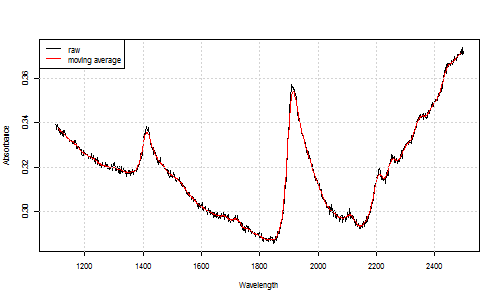### Savitzky-Golay filtering

Savitzky-Golay filtering [@savitzky1964] is a very common preprocessing technique. It fits a local polynomial regression on the signal and requires equidistant bandwidth. Mathematically, it operates simply as a weighted sum of neighbouring values:

$x_j\ast = \frac{1}{N}\sum_{h=-k}^{k}{c_hx_{j+h}}$

where $$x_j\ast$$ is the new value, $$N$$ is a normalizing coefficient, $$k$$ is the number of neighbour values at each side of $$j$$ and $$c_h$$ are pre-computed coefficients, that depends on the chosen polynomial order and degree (smoothing, first and second derivative).

# p = polynomial order w = window size (must be odd) m = m-th derivative (0 =
# smoothing) The function accepts vectors, data.frames or matrices.  For a matrix
# input, observations should be arranged row-wise
sgvec <- savitzkyGolay(X = NIRsoil$spc[1, ], p = 3, w = 11, m = 0) sg <- savitzkyGolay(X = NIRsoil$spc, p = 3, w = 11, m = 0)
# note that bands at the edges of the spectral matrix are lost !
dim(NIRsoil$spc) ##  825 700 dim(sg) ##  825 690  ## Derivatives Taking (numerical) derivatives of the spectra can remove both additive and multiplicative effects in the spectra and have other consequences as well (Table 2). Table 2. Pro's and con's of using derivative spectra. Advantage Drawback Reduce of baseline offset Risk of overfitting the calibration model Can resolve absorption overlapping Increase noise, smoothing required Compensates for instrumental drift Increase uncertainty in model coefficients Enhances small spectral absorptions Complicate spectral interpretation Often increase predictive accuracy Remove the baseline ! for complex datasets First and second derivatives of a spectrum can be computed with the finite difference method (difference between to subsequent data points), provided that the band width is constant: $x_i' = x_i - x_{i-1}$ $x_i'‘ = x_{i-1} - 2 \cdot x_i + x_{i+1}$ In R, this can be simply achieved with the diff function in base: # X = wavelength Y = spectral matrix n = order d1 <- t(diff(t(NIRsoil$spc), differences = 1))  # first derivative
d2 <- t(diff(t(NIRsoil$spc), differences = 2)) # second derivative plot(as.numeric(colnames(d1)), d1[1, ], type = "l", lwd = 1.5, xlab = "Wavelength", ylab = "") lines(as.numeric(colnames(d2)), d2[1, ], lwd = 1.5, col = "red") grid() legend("topleft", legend = c("1st der", "2nd der"), lty = c(1, 1), col = c("black", "red"))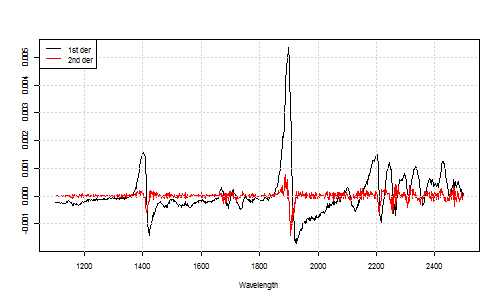One can see that derivatives tend to increase noise. One can use gap derivatives or the Savitzky-Golay algorithm to solve this. The gap derivative is computed simply as: $x_i’ = x_{i+k} - x_{i-k}$ $x_i'‘ = x_{i-k} - 2 \cdot x_i + x_{i+k}$ where $$k$$ is the gap size. Again, this can be easily achieved in R using the lag argument of the diff function # first derivative with a gap of 10 bands gd1 <- t(diff(t(NIRsoil$spc), differences = 1, lag = 10))


For more flexibility and control over the degree of smoothing, one could however use the Savitzky-Golay (savitzkyGolay) and Gap-segment derivative (gapDer) algorithms. The Gap-segment algorithms performs first a smoothing under a given segment size, followed by a derivative of a given order under a given gap size. Here is an exemple of the use of the gapDer function.

# m = order of the derivative w = window size ( = {2 * gap size} + 1) s = segment
# size first derivative with a gap of 10 bands
gsd1 <- gapDer(X = NIRsoil$spc, m = 1, w = 11, s = 10) plot(as.numeric(colnames(d1)), d1[1, ], type = "l", lwd = 1.5, xlab = "Wavelength", ylab = "") lines(as.numeric(colnames(gsd1)), gsd1[1, ], lwd = 1.5, col = "red") grid() legend("topleft", legend = c("1st der", "gap-segment 1st der"), lty = c(1, 1), col = c("black", "red"))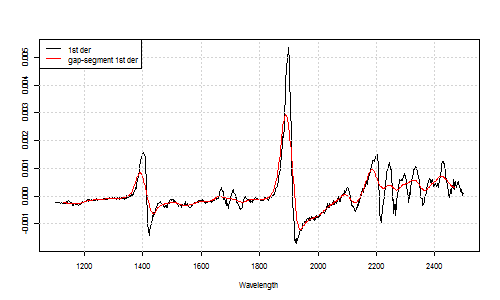## Scatter corrections Undesired spectral variations due to light scatter effects and variations in effective path length can be removed using scatter corrections. ### Standard Normal Variate (SNV) Standard Normal Variate (SNV) is another simple way for normalizing spectra that intends to correct for light scatter. It operates row-wise: $SNV_i = \frac{x_i - \bar{x_i}}{s_i}$ snv <- standardNormalVariate(X = NIRsoil$spc)


According to @fearn2008, it is better to perform SNV transformation after filtering (by e.g. Savitzky-Golay) than the reverse.

### SNV-Detrend

The SNV-Detrend [@barnes1989] further accounts for wavelength-dependent scattering effects (variation in curvilinearity between the spectra). After a SNV transformation, a 2$$^{nd}$$-order polynomial is fit to the spectrum and subtracted from it.

# X = input spectral matrix wav = band centers
dt <- detrend(X = NIRsoil$spc, wav = as.numeric(colnames(NIRsoil$spc)))
plot(NIRsoil$spc[1, ], type = "l", xlab = "Band number", ylab = "", lwd = 1.5) par(new = TRUE) plot(dt[1, ], xaxt = "n", yaxt = "n", xlab = "", ylab = "", lwd = 1.5, col = "red", type = "l") axis(4, col = "red") grid() legend("topleft", legend = c("raw", "detrend signal"), lty = c(1, 1), col = c("black", "red"))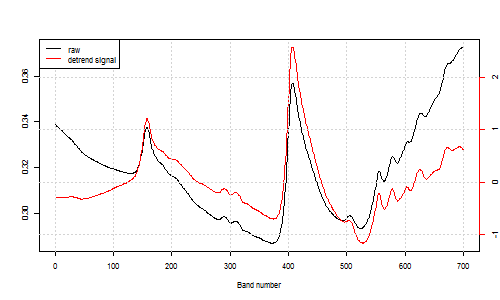par(new = FALSE)  ## Centering and scaling Centering and scaling tranforms a given matrix to a matrix with columns with zero mean (centering), unit variance (scaling) or both (auto-scaling): $Xc_{ij} = X_{ij} - \bar{X}_{j}$ $Xs_{ij} = \frac{X_{ij} - \bar{X}_{j}}{s_{j}}$ where $$Xc$$ and $$Xs$$ are the mean centered and auto-scaled matrices, $$X$$ is the input matrix, $$\bar{X}_{j}$$ and $$s_{j}$$ are the mean and standard deviation of variable $$j$$. In R, these operations are simply obtained with the scale function. Other types of scaling can be considered. Spectroscopic models can often be improved by using ancillary data (e.g. temperature, …) [@fearn2010]. Due to the nature of spectral data (multivariate), other data would have great chance to be dominated by the spectral matrix and have no chance to contribute significantly to the model due to purely numerical reasons [@eriksson2006]. One can use block scaling to overcome this limitation. It basically uses different weights for different block of variables. With soft block scaling, each block is scaled (ie each column divided by a factor) such that the sum of their variance is equal to the square root of the number of variables in the block. With hard block scaling, each block is scaled such that the sum of their variance is equal to 1. # X = spectral matrix type = 'soft' or 'hard' The ouptut is a list with the # scaled matrix (Xscaled) and the divisor (f) bs <- blockScale(X = NIRsoil$spc, type = "hard")$Xscaled sum(apply(bs, 2, var)) # this works! ##  1  he problem with block scaling is that it down-scale all the block variables to the same variance. Since sometimes this is not advised, one can alternatively use sum of squares block weighting . The spectral matrix is multiplied by a factor to achieve a pre-determined sum of square: # X = spectral matrix targetnorm = desired norm for X bn <- blockNorm(X = NIRsoil$spc, targetnorm = 1)$Xscaled sum(bn^2) # this works! ##  1  ## Other transformations ### Continuum removal The continuum removal technique was introduced by @clark1984 as an effective method to highlight absorption features of minerals. It can be viewed as an albedo normalization technique. This technique is based on the computation of the continuum (or envelope) of a given spectrum. The continuum-removed spectrum of a given spectrum is computed as follows: 1. The convex hull points are identified. 2. Then, these points are connected by linear interpolation to form the continuum. 3. The continuum-removed spectrum is given by $$\phi_{i} = \frac{x_{i}}{c_{i}}; i=\left \{ 1,…, p\right\}$$, where $$x_{i}$$ and $$c_{i}$$ are the original and the continuum reflectance (or absorbance) values respectively at the $$i$$th wavelength of a set of $$p$$ wavelengths, and $$\phi_{i}$$ is the final reflectance (or absorbance) value after continuum removal. The continuumRemoval function allows to compute the continuum-removed values of either reflectance or absorbance spectra. # type of data: 'R' for reflectance (default), 'A' for absorbance cr <- continuumRemoval(X = NIRsoil$spc, type = "A")
# plot of the 10 first abs spectra
matplot(as.numeric(colnames(NIRsoil$spc)), t(NIRsoil$spc[1:3, ]), type = "l", lty = 1,
ylim = c(0, 0.6), xlab = "Wavelength /nm", ylab = "Absorbance")
matlines(as.numeric(colnames(NIRsoil$spc)), lty = 1, t(cr[1:3, ])) grid()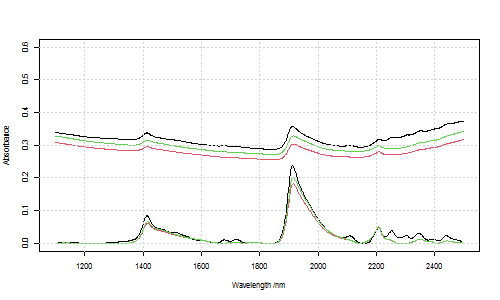### Resampling To match the response of one instrument with another, a signal can be resampled to new band positions by simple interpolation (resample) or using full width half maximum (FWHM) values (resample2). # Calibration sampling algorithms Calibration models are usually developed on a representative portion of the data (training set) and validated on the remaining set of samples (test/validation set). There are several solutions for selecting samples, e.g.: • random selection (see e.g. sample function in base) • stratified random sampling on percentiles of the response $$y$$ • use the spectral data. For selecting representative samples, the prospect package provides functions that use the third solution. The following functions are available: • naes: k-means Sampling [@naes2002] • kenStone: Kennard-Stone Sampling a.k.a. CADEX Sampling [@kennard1969] • duplex: Duplex Sampling [@snee1977] • puchwein: Factor Analysis Sampling [@puchwein1988] • shenkWest: SELECT Sampling [@shenk1991] • honigs: Honigs Sampling [@honigs1985] ### $$k$$-means sampling (naes) The $$k$$-means sampling simply uses $$k$$-means clustering algorithm. To sample a subset of $$n$$ samples $$X_{tr} = \left \{ {x_{tr}}_{j} \right \}_{j=1}^{n}$$, from a given set of $$N$$ samples $$X = \left \{ x_i \right \}_{i=1}^{N}$$ (note that $$N>n$$) the algorithm works as follows: 1. Perform a $$k$$-means clustering of $$X$$ using $$n$$ clusters. 2. Extract the $$n$$ centroids ($$c$$, or prototypes). This can be also the sample that is the farthest away from the centre of the data, or a random selection. See the method argument in naes 3. Calculate the distance of each sample to each $$c$$. 4. For each $$c$$ allocate in $$X_{tr}$$ its closest sample found in $$X$$. # X = the input matrix k = number of calibration samples to be selected pc = if # pc is specified, k-mean is performed in the pc space (here we will use only the # two 1st pcs) iter.max = maximum number of iterations allowed for the k-means # clustering. kms <- naes(X = NIRsoil$spc, k = 5, pc = 2, iter.max = 100)
# Plot the pcs scores and clusters
plot(kms$pc, col = rgb(0, 0, 0, 0.3), pch = 19, main = "k-means") grid() # Add the selected points points(kms$pc[kms$model, ], col = "red", pch = 19)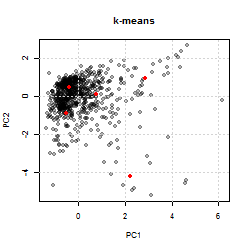### Kennard-Stone sampling (kenStone) To sample a subset of $$n$$ samples $$X_{tr} = \left \{ {x_{tr}}_{j} \right \}_{j=1}^{n}$$, from a given set of $$N$$ samples $$X = \left \{ x_i \right \}_{i=1}^{N}$$ (note that $$N>n$$) the Kennard-Stone (CADEX) sampling algorithm consists in @kennard1969: 1. Find in $$X$$ the samples $${x_{tr}}_1$$ and $${x_{tr}}_2$$ that are the farthest apart from each other, allocate them in $$X_{tr}$$ and remove them from $$X$$. 2. Find in $$X$$ the sample $${x_{tr}}_3$$ with the maximum dissimilarity to $$X_{tr}$$. Allocate $${x_{tr}}_3$$ in $$X_{tr}$$ and then remove it from $$X$$. The dissimilarity between $$X_{tr}$$ and each $$x_i$$ is given by the minimum distance of any sample allocated in $$X_{tr}$$ to each $$x_i$$. In other words, the selected sample is one of the nearest neighbours of the points already selected which is characterized by the maximum distance to the other points already selected. 3. Repeat the step 2 n-3 times in order to select the remaining samples ($${x_{tr}}_4,…, {x_{tr}}_n$$). The Kennard-Stone algorithm allows to create a calibration set that has a flat distribution over the spectral space. The metric used to compute the distance between points can be either the Euclidean distance or the Mahalanobis distance. One of the drawbacks of this algorithm is that it is prone to outlier selection [@ramirez2014], therefore outlier analysis is recommended before sample selction. Let's see some examples… # Create a dataset for illustrating how the calibration sampling algorithms work X <- data.frame(x1 = rnorm(1000), x2 = rnorm(1000)) plot(X, col = rgb(0, 0, 0, 0.3), pch = 19, main = "Kennard-Stone (synthetic)") grid() # kenStone produces a list with row index of the points selected for calibration ken <- kenStone(X, k = 40) points(X[ken$model, ], col = "red", pch = 19, cex = 1.4)  # plot selected points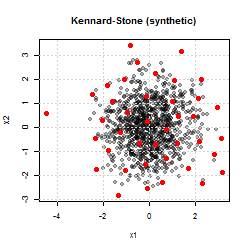# Test with the NIRsoil dataset one can also use the mahalanobis distance (metric
# argument) computed in the pc space (pc argument)
plot(pu$pc, col = rgb(0, 0, 0, 0.3), pch = 19, main = "puchwein") grid() points(pu$pc[pu$model, ], col = "red", pch = 19) # selected samples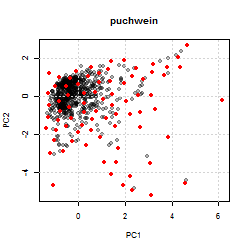par(mfrow = c(2, 1)) plot(pu$leverage$removed, pu$leverage$diff, type = "l", xlab = "# samples removed", ylab = "Difference between th. and obs sum of leverages") # This basically shows that the first loop is optimal plot(pu$leverage$loop, nrow(NIRsoil) - pu$leverage$removed, xlab = "# loops", ylab = "# samples kept", type = "l") par(mfrow = c(1, 1))  ### Honigs (honigs) The Honigs algorithm selects samples based on the size of their absorption features [@honigs1985]. It can works both on absorbance and continuum-removed spectra. The sample having the highest absorption feature is selected first. Then this absorption is substracted from other spectra and the algorithm iteratively select samples with the highest absorption (in absolute value) until the desired number of samples is reached. ho <- honigs(X = NIRsoil$spc, k = 10, type = "A")  # type = 'A' is for absorbance data
# plot calibration spectra
matplot(as.numeric(colnames(NIRsoil$spc)), t(NIRsoil$spc[ho$model, ]), type = "l", xlab = "Wavelength", ylab = "Absorbance") # add bands used during the selection process abline(v = as.numeric(colnames(NIRsoil$spc))[ho\$bands], lty = 2)